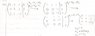# Question about solving augmented matrices and row operations

• TheColorCute

#### TheColorCute

So I just started my Linear Algebra course yesterday. I am confused on one aspect. When asked to solve an augmented matrix, the teacher would employ row operations. I understand how the row operations lead from one matrix to the next, but what I don't understand is how we formulate which row operation to use. The teacher would write down R2=R2+4R3 or something and I don't understand how he came up with this. See picture:

For instance, in the picture, to get from the first matrix to the second matrix he said that R2=R2-2R1 and that R3=R3-R1. How does he come up with this? I'm lost eheh...

Basically, I don't understand how he gets from the matrix to the row operations. Can someone please explain it to me?

Thanks! :)

#### Attachments

•LA_question_crop.jpg
17.2 KB · Views: 286

So I just started my Linear Algebra course yesterday. I am confused on one aspect. When asked to solve an augmented matrix, the teacher would employ row operations. I understand how the row operations lead from one matrix to the next, but what I don't understand is how we formulate which row operation to use. The teacher would write down R2=R2+4R3
This means replace row 2 by row 2 + 4 times row3. IOW, add 4 times row 3 to row 2, replacing row 2.
or something and I don't understand how he came up with this. See picture:

For instance, in the picture, to get from the first matrix to the second matrix he said that R2=R2-2R1
Replace row 2 by itself minus 2 times row 1.
and that R3=R3-R1. How does he come up with this? I'm lost eheh...

Basically, I don't understand how he gets from the matrix to the row operations. Can someone please explain it to me?

Thanks! :)

Well, I understand what they mean. I don't understand how we know that in order to get R2 in the second matrix we have to add R2+4R3 from the first matrix. How do we know what to add, subtract, multiply, or divide? How do we know that R2=R2+4R3. That's what I'm confused about.

Last edited:
It wasn't clear to me that you understood what they meant. In the augmented matrix in the thumbnail, your instructor wants to use the leading entry of row 1 to eliminate the leading entries in rows 2 and 3. To do this he added -2 times row 1 to row 2 and -1 times row 1 to row 3. The result of that was 0 entries in the first column of rows 2 and 3.

Well, I understand what they mean. I don't understand how we know that in order to get R2 in the second matrix we have to add R2+4R3 from the first matrix. How do we know what to add, subtract, multiply, or divide? How do we know that R2=R2+4R3. That's what I'm confused about.

R2=R2+4R3 is not saying that row 2 is equal to row 2 + 4*row3. It is merely notation indicating what he plans to do - it's a form of bookkeeping.

Ohhhh OK. So it's sort of like solving a simple system of linear equations by the elimination method, right?
So I suppose my next question is how do we know when to stop simplifying the matrices?
I see in the final matrix that the third row is completely eliminated (it's all zeros).
Also, how do we know was x1, x2, and x3 are?

When to stop depends on whether you want to end with echelon form or reduced, row-echelon form. Your book should define these terms.

In your problem the augemented matrix was taken almost all the way to reduced, row-echelon form. I say almost, because the 2nd row could be replaced by -1 times itself. This would give you
[1 1 0 | 1]
[0 0 1 | -1]
[0 0 0 | 0]
The numbers on the left side of the augmented matrix represent the coefficients of the variables. The numbers on the right side represent the constants. The RREF matrix just above says
1x1 + 1x2 = 1
1x3 = -1

(I put the coefficients in just for emphasis.)

In the system above, I could solve for x1 in terms of x2, which would make x2 arbitrary.

IOW, we could say
x1 = -x2 + 1
x2 = x2
x3 = -1

OK, so it appears that each coefficient on the left side of the matrix gets it's own variable (x1, x2, x3, etc.) So if we had 6 coefficients in the matrix, we'd have variables x1->6? For example, given the matrix:

[2 3 4 | 1]
[1 0 7 | 2]
[0 0 5 | 3]

we'd say that 2x1+3x2+4x3=1, 1x4+7x5=2, and finally 5x6=3?

And from there if we wanted to we could solve for x1 in terms of x2 and x3 in the 2x1+3x2+4x3=1 equation?
And that would work out to be x1=(1/2)(-3x2-4x3+1)?

No, not at all. Each column of the matrix on the left is associated with one variable, so the variables are just x1, x2, and x3.

Then what would happen if we had two non-zero coefficients in the same column? Like,

[2 1 | 1]
[3 0 | 2]

Would that be 2x1+1x2=1 and 3x1+0x2=2??

Yes.

Yes.

Oh OK. Thanks so much for your help! :)